Скачать презентацию State space model of precipitation rate Tamre Cardoso

a7fcb4892ee8eb1a21c835f7130cb30e.ppt

• Количество слайдов: 24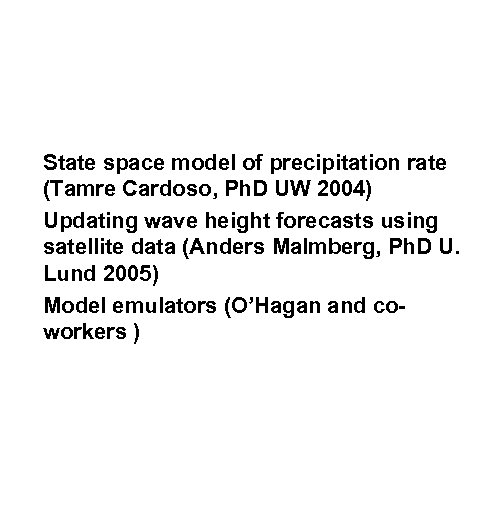State space model of precipitation rate (Tamre Cardoso, Ph. D UW 2004) Updating wave height forecasts using satellite data (Anders Malmberg, Ph. D U. Lund 2005) Model emulators (O’Hagan and coworkers )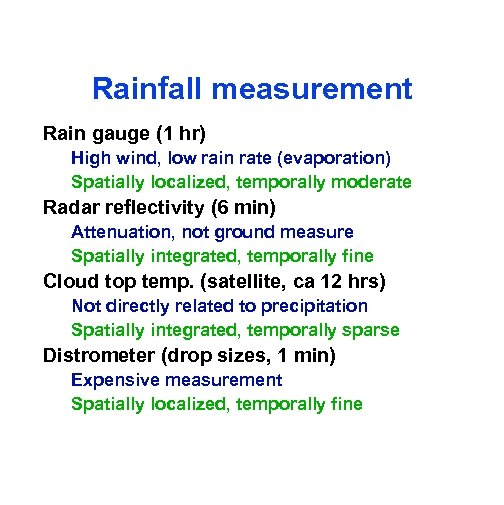Rainfall measurement Rain gauge (1 hr) High wind, low rain rate (evaporation) Spatially localized, temporally moderate Radar reflectivity (6 min) Attenuation, not ground measure Spatially integrated, temporally fine Cloud top temp. (satellite, ca 12 hrs) Not directly related to precipitation Spatially integrated, temporally sparse Distrometer (drop sizes, 1 min) Expensive measurement Spatially localized, temporally fine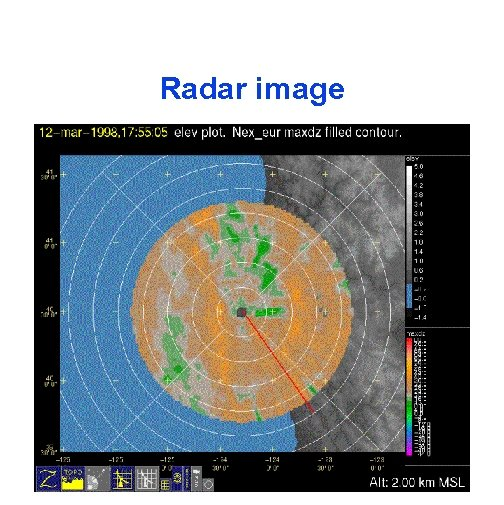Radar image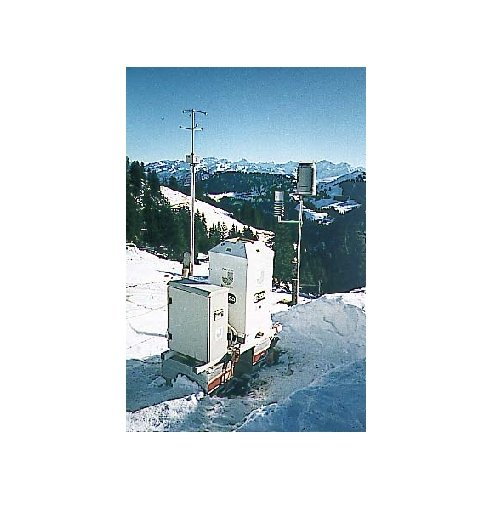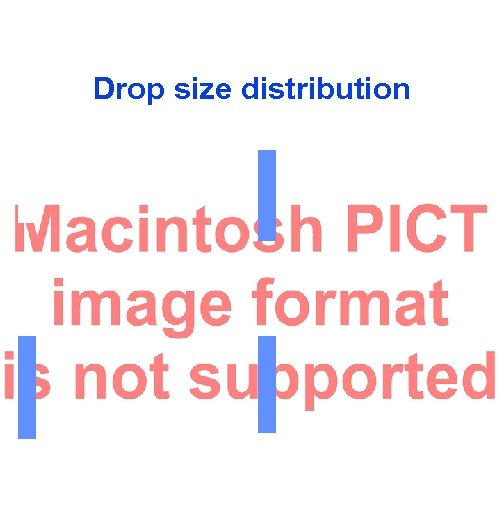Drop size distribution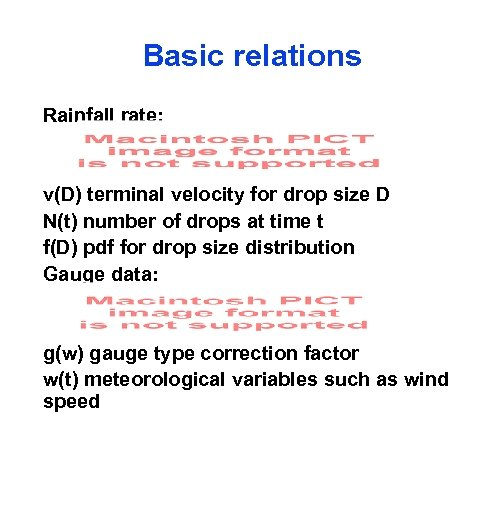Basic relations Rainfall rate: v(D) terminal velocity for drop size D N(t) number of drops at time t f(D) pdf for drop size distribution Gauge data: g(w) gauge type correction factor w(t) meteorological variables such as wind speed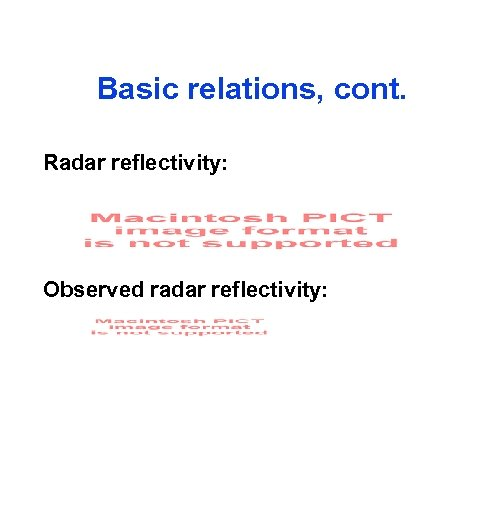Basic relations, cont. Radar reflectivity: Observed radar reflectivity: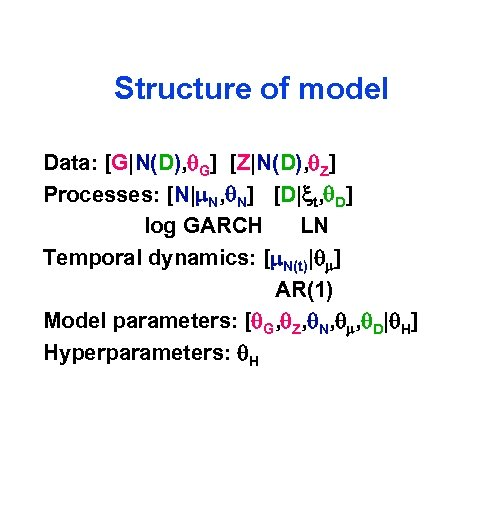Structure of model Data: [G|N(D), q. G] [Z|N(D), q. Z] Processes: [N|m. N, q. N] [D|xt, q. D] log GARCH LN Temporal dynamics: [m. N(t)|qm] AR(1) Model parameters: [q. G, q. Z, q. N, qm, q. D|q. H] Hyperparameters: q. H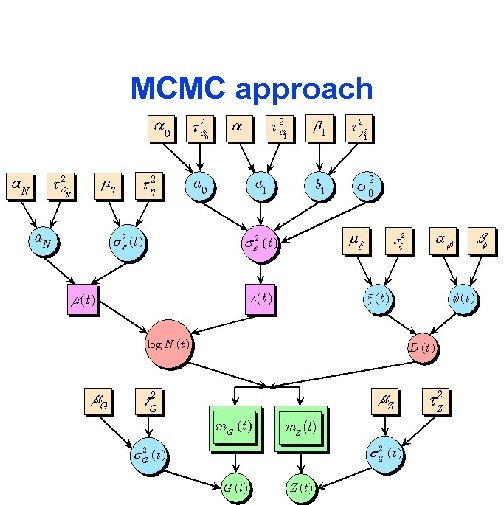MCMC approach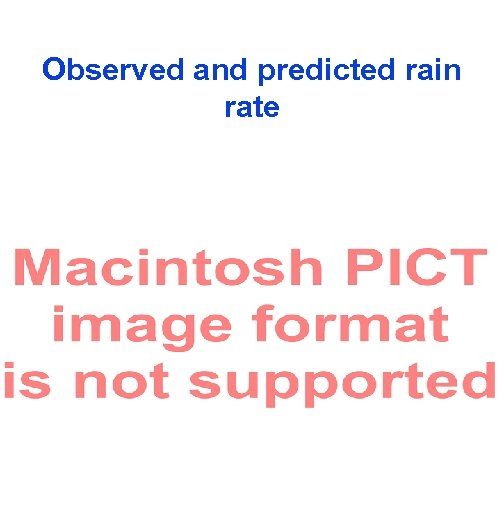Observed and predicted rain rate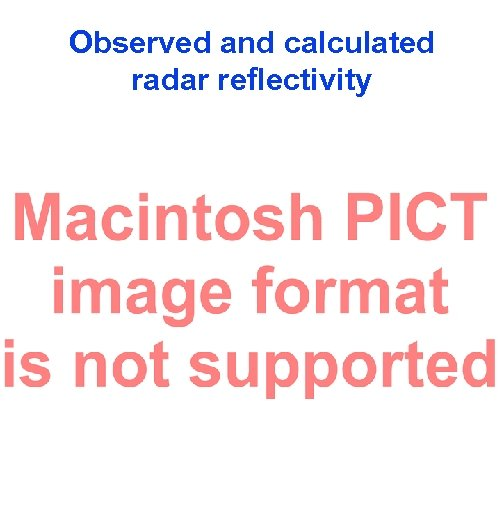Observed and calculated radar reflectivity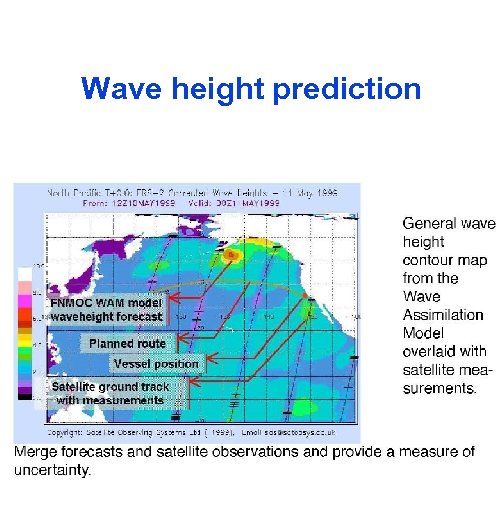Wave height prediction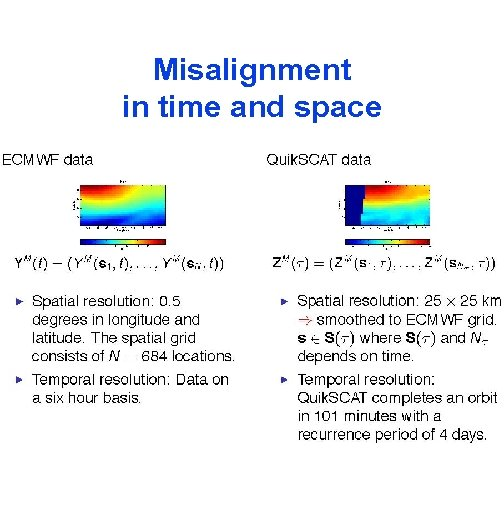Misalignment in time and space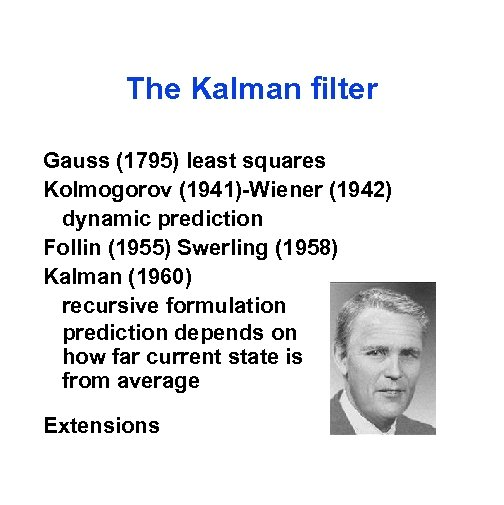The Kalman filter Gauss (1795) least squares Kolmogorov (1941)-Wiener (1942) dynamic prediction Follin (1955) Swerling (1958) Kalman (1960) recursive formulation prediction depends on how far current state is from average Extensions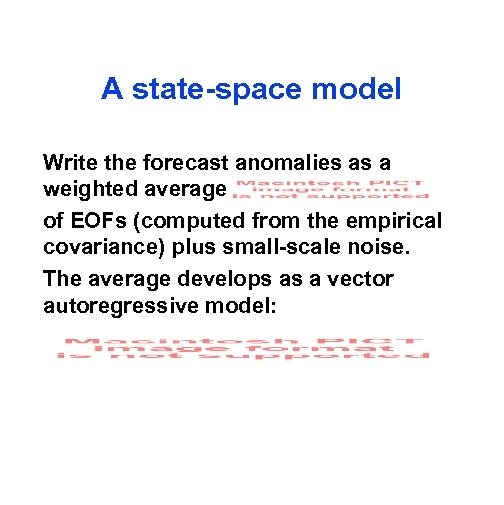A state-space model Write the forecast anomalies as a weighted average of EOFs (computed from the empirical covariance) plus small-scale noise. The average develops as a vector autoregressive model: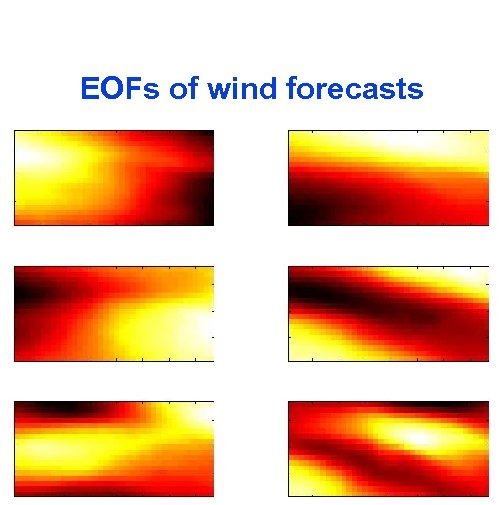EOFs of wind forecasts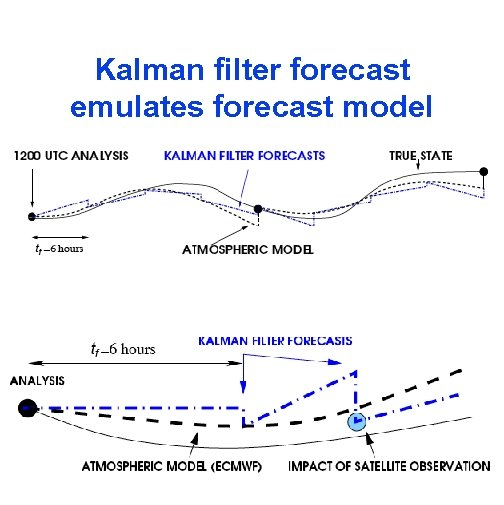Kalman filter forecast emulates forecast model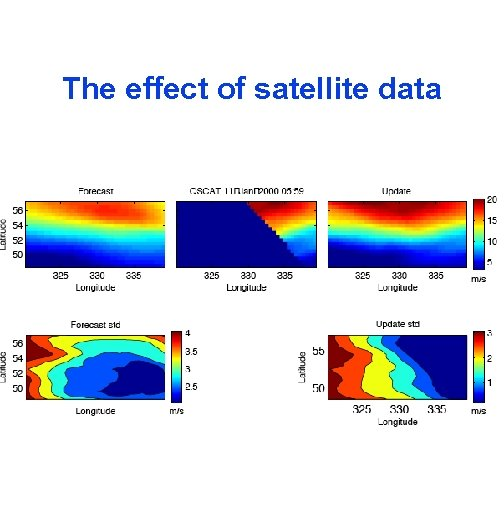The effect of satellite data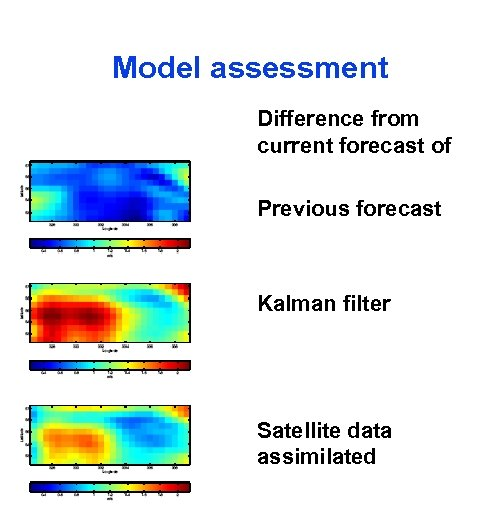Model assessment Difference from current forecast of Previous forecast Kalman filter Satellite data assimilated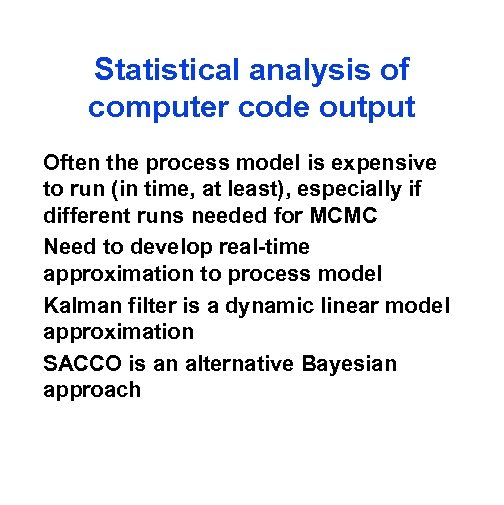Statistical analysis of computer code output Often the process model is expensive to run (in time, at least), especially if different runs needed for MCMC Need to develop real-time approximation to process model Kalman filter is a dynamic linear model approximation SACCO is an alternative Bayesian approach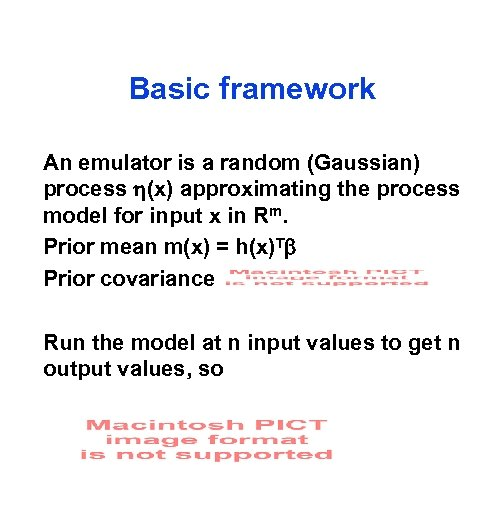Basic framework An emulator is a random (Gaussian) process (x) approximating the process model for input x in Rm. Prior mean m(x) = h(x)T Prior covariance Run the model at n input values to get n output values, so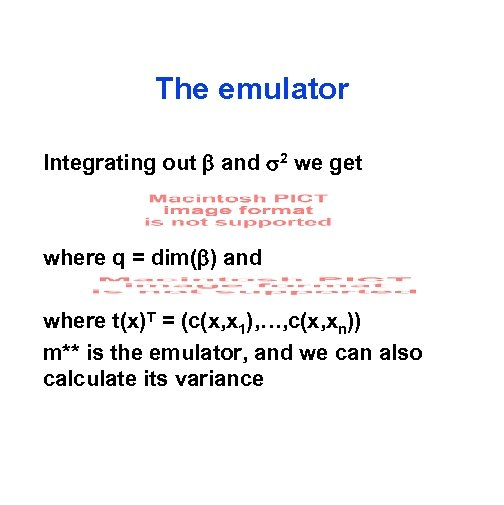The emulator Integrating out and 2 we get where q = dim( ) and where t(x)T = (c(x, x 1), …, c(x, xn)) m** is the emulator, and we can also calculate its variance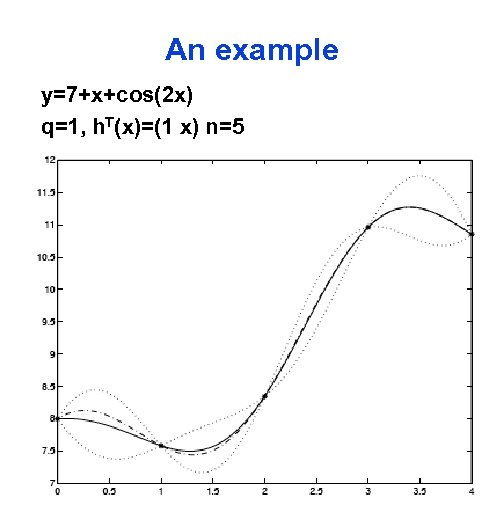An example y=7+x+cos(2 x) q=1, h. T(x)=(1 x) n=5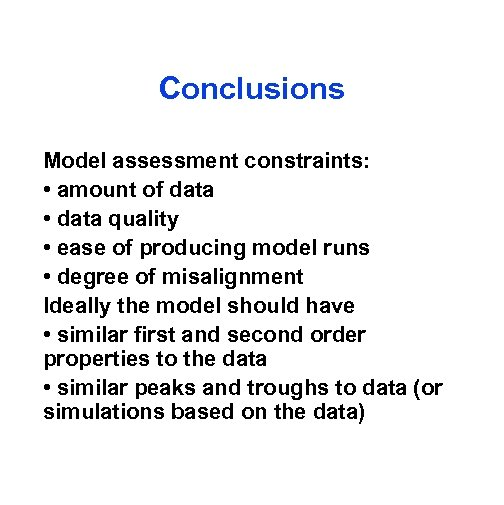Conclusions Model assessment constraints: • amount of data • data quality • ease of producing model runs • degree of misalignment Ideally the model should have • similar first and second order properties to the data • similar peaks and troughs to data (or simulations based on the data)